• 二、QuickSort.h #include<iostream> using namespace std; template<class T> class QuickSort { private: T a = {'d','a','f','c','v','b','a','g','s','z'}; int size...
一、结果显示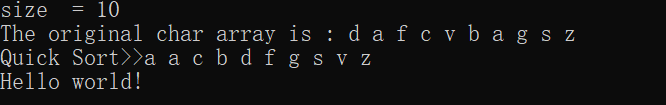二、QuickSort.h
#include<iostream>

using namespace std;

template<class T>
class QuickSort
{
private:
T a = {'d','a','f','c','v','b','a','g','s','z'};
int size = sizeof(a);
public:
QuickSort()
{
cout<<"size  = "<<size<<endl;
}

void QuickSort00()
{
T b;
for(int i = 0; i < size; i++)
b[i] = a[i];
QuickSort0( b, 0, size-1);
cout<<"Quick Sort>>";
print(b);
}

void QuickSort0(T a[], int left,int right )
{
if(left < right)
{
T pivot = median( a, left, right);
int i = left,j = right-1;
for( ; ; )
{
while(a[++i] < pivot) {}
while(pivot < a[--j]) {}
if(i < j)        //Repeat until i > j ;
swap(a[i], a[j]);
else
break;
}
swap(a[i], a[right-1]);       //The bigger at Position "i" !!!
QuickSort0( a, left, i-1);
QuickSort0( a, i+1, right);
}

}

const T & median(T a[], int left,int right)
{
int center = (left + right)/2;
if(a[left] > a[center])
swap(a[left], a[center]);
if(a[center] > a[right])
swap(a[center], a[right]);
if(a[left] > a[right])
swap(a[right], a[left]);
swap(a[center], a[right-1]);    //Place the pivot at right-1 , the right is biggest !!
return a[right-1];
}

void vprint()
{
cout<<"The original char array is : ";
print(a);
}
void print(char a)
{
for(int i = 0 ; i < size ; i++)
{
cout<<a[i]<<" ";
}
cout<<endl;
}
};


三、main.cpp
#include "QuickSort.h"

using namespace std;

int main()
{
QuickSort<char> T;
T.vprint();
T.QuickSort00();
cout << "Hello world!" << endl;
return 0;
}


展开全文• 在这里插入代码片 #include<stdio.h> int part(int a[],int ...void quicksort(int a[],int p,int r) { int q; if(p<r) { q=part(a,p,r); quicksort(a,p,q-1); quicksort(a,q+1,r); } } int p...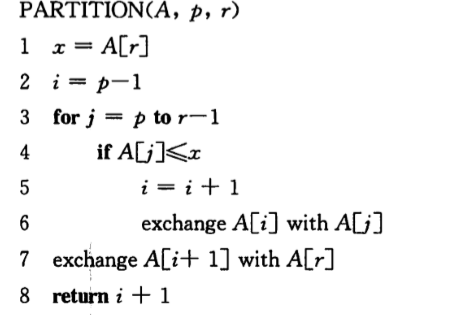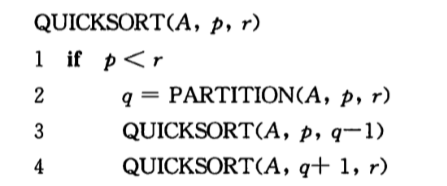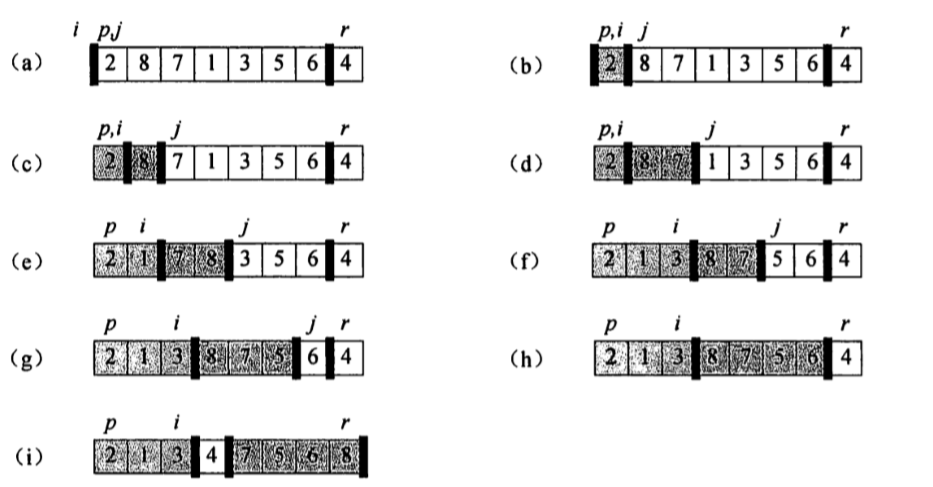在这里插入代码片
#include<stdio.h>
int part(int a[],int p,int r);
void quicksort(int a[],int p,int r)
{
int q;
if(p<r)
{
q=part(a,p,r);
quicksort(a,p,q-1);
quicksort(a,q+1,r);
}
}
int part(int a[],int p,int r)
{
int x,i,t,j;
i=p-1;
x=a[r];

for(j=p;j<r;j++)
{
if(a[j]<=x)
{
i++;
t=a[i];
a[i]=a[j];
a[j]=t;

}
}
t=a[i+1];
a[i+1]=a[r];
a[r]=t;
return i+1;
}
int main()
{
int a={2,8,7,1,3,5,6,4};
int i;
quicksort(a,0,7);
for(i=0;i<8;i++)
{
printf("%3d",a[i]);
}

}

图中的partition即代码中的part函数是为了达到a[q]>a[i],i<q; a[q]<a[j],j>q;是为了把a[r]放到中间
展开全文• public class test3 { public static void main(String[] args) { int[] arr = { 49, 38, 65, 97, 23, 22, 76, 1, 5, 8, 2, 0, -1, 22 }; quickSort(arr, 0, arr.length - 1); Sy...


public class test3 {
public static void main(String[] args) {
int[] arr = { 49, 38, 65, 97, 23, 22, 76, 1, 5, 8, 2, 0, -1, 22 };
quickSort(arr, 0, arr.length - 1);
System.out.println("排序后:");
for (int i : arr) {
System.out.println(i);
}
}

public static void quickSort(int[] arr,int low,int hight){
int left = low;
int right = hight;
if (left < right) {
int temp = arr[left];
while (left < right) {
while (left < right && arr[right] >= temp) {
right--;
}
arr[left] = arr[right];
while (left < right && arr[left] <= temp) {
left++;
}
arr[right] = arr[left];
}
arr[left] = temp;
quickSort(arr, low, left - 1);
quickSort(arr, left + 1, hight);
}
}
}


展开全文• Quicksort Quicksort is a fast sorting algorithm, which is used not only for educational purposes, but widely applied in practice. On the average, it has O(n log n) complexity, making quicksort suitab
Quicksort
Quicksort is a fast sorting algorithm, which is used not only for educational purposes, but widely applied in practice. On the average, it has O(n log n) complexity, making quicksort suitable for sorting big data volumes. The idea of the algorithm is quite simple and once you realize it, you can write quicksort as fast as bubble sort.
Algorithm The divide-and-conquer strategy is used in quicksort. Below the recursion step is described:
Choose a pivot value. We take the value of the middle element as pivot value, but it can be any value, which is in range of sorted values, even if it doesn't present in the array.Partition. Rearrange elements in such a way, that all elements which are lesser than the pivot go to the left part of the array and all elements greater than the pivot, go to the right part of the array. Values equal to the pivot can stay in any part of the array. Notice, that array may be divided in non-equal parts.Sort both parts. Apply quicksort algorithm recursively to the left and the right parts.
Partition algorithm in detail
There are two indices i and j and at the very beginning of the partition algorithm i points to the first element in the array and j points to the last one. Then algorithm moves iforward, until an element with value greater or equal to the pivot is found. Index j is moved backward, until an element with value lesser or equal to the pivot is found. If i ≤ j then they are swapped and i steps to the next position (i + 1), j steps to the previous one (j - 1). Algorithm stops, when i becomes greater than j.
After partition, all values before i-th element are less or equal than the pivot and all values after j-th element are greater or equal to the pivot.
Example. Sort {1, 12, 5, 26, 7, 14, 3, 7, 2} using quicksort.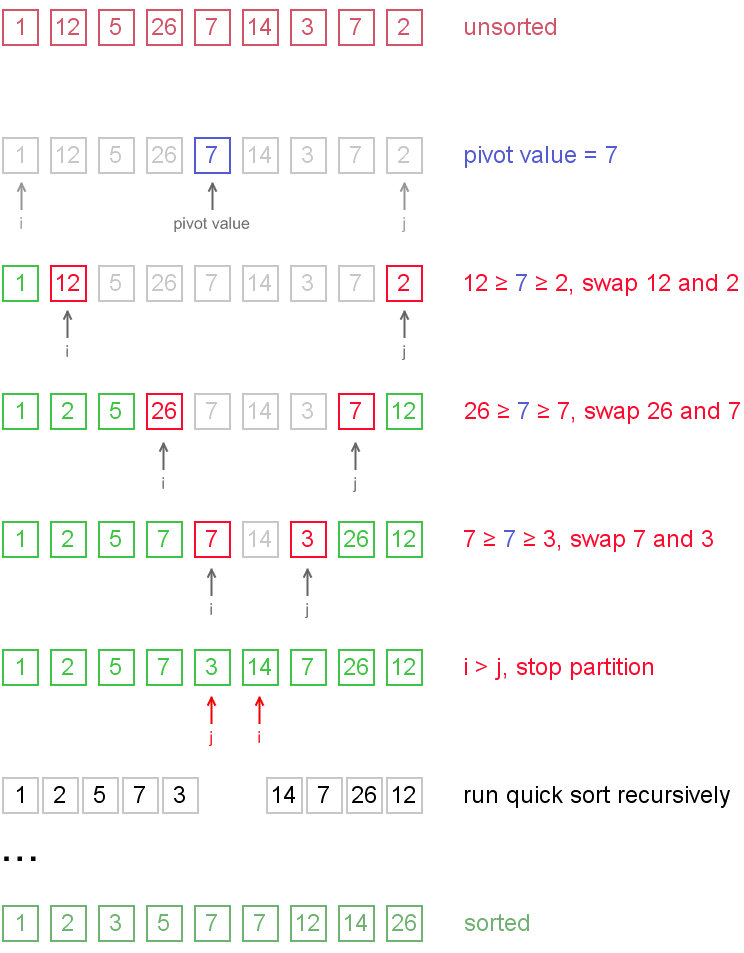Notice, that we show here only the first recursion step, in order not to make example too long. But, in fact, {1, 2, 5, 7, 3} and {14, 7, 26, 12} are sorted then recursively.
Why does it work? On the partition step algorithm divides the array into two parts and every element
a from the left part is less or equal than every element
b from the right part. Also
a and
bsatisfy
a ≤ pivot ≤ b inequality. After completion of the recursion calls both of the parts become sorted and, taking into account arguments stated above,
the whole array is sorted.

Complexity analysis
On the average quicksort has O(n log n) complexity, but strong proof of this fact is not trivial and not presented here. Still, you can find the proof in . In worst case, quicksort runs O(n2) time, but on the most "practical" data it works just fine and outperforms other O(n log n) sorting algorithms.
Code snippets
Partition algorithm is important per se, therefore it may be carried out as a separate function. The code for C++ contains solid function for quicksort, but Java code contains two separate functions for partition and sort, accordingly.
Java
int partition(int arr[], int left, int right)
{
int i = left, j = right;
int tmp;
int pivot = arr[(left + right) / 2];

while (i <= j) {
while (arr[i] < pivot)
i++;
while (arr[j] > pivot)
j--;
if (i <= j) {
tmp = arr[i];
arr[i] = arr[j];
arr[j] = tmp;
i++;
j--;
}
};

return i;
}

void quickSort(int arr[], int left, int right) {
int index = partition(arr, left, right);
if (left < index - 1)
quickSort(arr, left, index - 1);
if (index < right)
quickSort(arr, index, right);
}
C++
void quickSort(int arr[], int left, int right) {
int i = left, j = right;
int tmp;
int pivot = arr[(left + right) / 2];

/* partition */
while (i <= j) {
while (arr[i] < pivot)
i++;
while (arr[j] > pivot)
j--;
if (i <= j) {
tmp = arr[i];
arr[i] = arr[j];
arr[j] = tmp;
i++;
j--;
}
};

/* recursion */
if (left < j)
quickSort(arr, left, j);
if (i < right)
quickSort(arr, i, right);
}
Full quicksort package Full quicksort package includes:
full quicksort package.
Recommended books
Cormen, Leiserson, Rivest. Introduction to algorithms. (Theory)Aho, Ullman, Hopcroft. Data Structures and Algorithms. (Theory)Robert Lafore. Data Structures and Algorithms in Java. (Practice)Mark Allen Weiss. Data Structures and Problem Solving Using C++. (Practice)
Visualizers
Quicksort Animation (with source code line by line visualization)Quicksort in Java Applets CentreAnimated Sorting Algorithms: Quicksort

Reference: http://www.algolist.net/Algorithms/Sorting/Quicksort

展开全文...